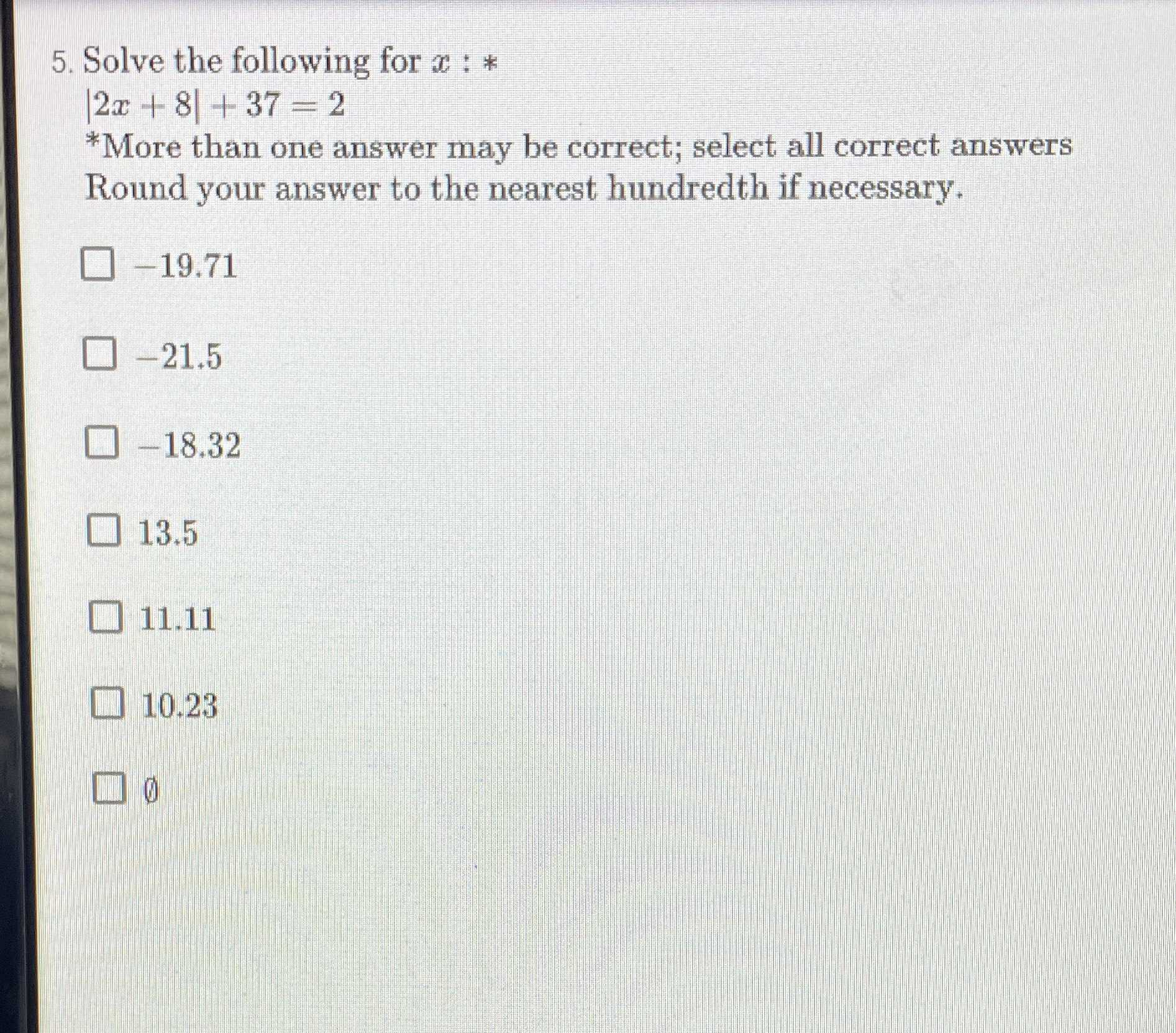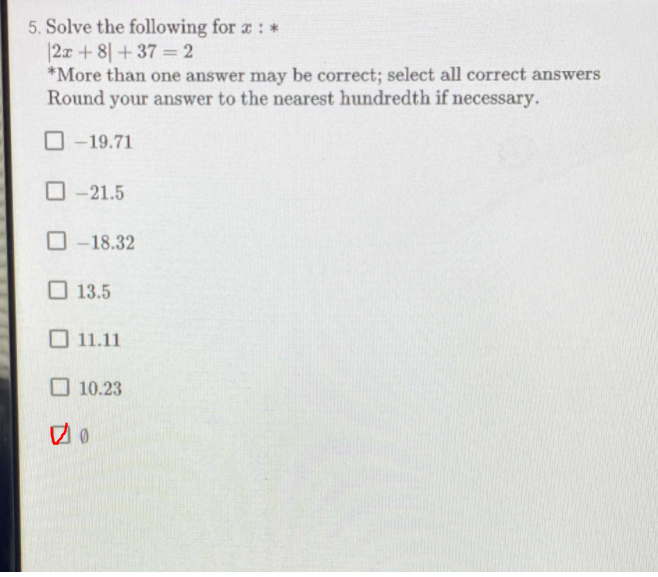### Still have math questions?

Algebra
Question5. Solve the following for $$x : *$$

$$| 2 x + 8 | + 37 = 2$$

$$\square - 19.71$$

$$\square - 21.5$$

$$\square - 18.32$$

$$\square 13.5$$

$$\square 10.23$$

$$\square 0$$